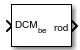# Direction Cosine Matrix to Rodrigues

Convert direction cosine matrix to Euler-Rodrigues vector

• Library:
• Aerospace Blockset / Utilities / Axes Transformations

•## Description

The Direction Cosine Matrix to Rodrigues block determines the 3-by-3 direction cosine matrix from a three-element Euler-Rodrigues vector. The rotation used in this block is a passive transformation between two coordinate systems. For more information on the direction cosine matrix, see Algorithms.

## Ports

### Input

expand all

Direction cosine matrix, specified as a 3-by-3 matrix, from which to determine the Euler-Rodrigues vector.

Data Types: `double`

### Output

expand all

Euler-Rodrigues vector, returned as a three-element vector.

Data Types: `double`

## Parameters

expand all

Block behavior when direction cosine matrix is invalid (not orthogonal).

• `Warning` — Displays warning and indicates that the direction cosine matrix is invalid.

• `Error` — Displays error and indicates that the direction cosine matrix is invalid.

• `None` — Does not display warning or error (default).

#### Programmatic Use

 Block Parameter: `action` Type: character vector Values: `'None'` | `'Warning'` | `'Error'` Default: `'None'`

Data Types: `char` | `string`

Tolerance of direction cosine matrix validity, specified as a scalar. The block considers the direction cosine matrix valid if these conditions are true:

• The transpose of the direction cosine matrix times itself equals `1` within the specified tolerance (`transpose(n)*n == 1±tolerance`)

• The determinant of the direction cosine matrix equals `1` within the specified tolerance (`det(n) == 1±tolerance`).

#### Programmatic Use

 Block Parameter: `tolerance` Type: character vector Values: `'eps(2)'` | scalar Default: `'eps(2)'`

Data Types: `double`

## Algorithms

An Euler-Rodrigues vector $\stackrel{⇀}{b}$ represents a rotation by integrating a direction cosine of a rotation axis with the tangent of half the rotation angle as follows:

`$\stackrel{\to }{b}=\left[\begin{array}{ccc}{b}_{x}& {b}_{y}& {b}_{z}\end{array}\right]$`

where:

`$\begin{array}{l}{b}_{x}=\mathrm{tan}\left(\frac{1}{2}\theta \right){s}_{x},\\ {b}_{y}=\mathrm{tan}\left(\frac{1}{2}\theta \right){s}_{y},\\ {b}_{z}=\mathrm{tan}\left(\frac{1}{2}\theta \right){s}_{z}\end{array}$`

are the Rodrigues parameters. Vector $\stackrel{⇀}{s}$ represents a unit vector around which the rotation is performed. Due to the tangent, the rotation vector is indeterminate when the rotation angle equals ±pi radians or ±180 deg. Values can be negative or positive.

 Dai, J.S. "Euler-Rodrigues formula variations, quaternion conjugation and intrinsic connections." Mechanism and Machine Theory, 92, 144-152. Elsevier, 2015.